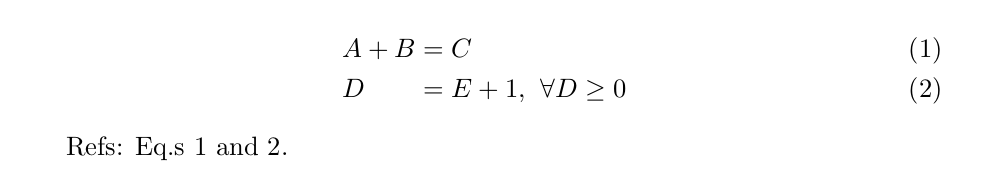# Latex math: align at multiple places -- alignat

latex

It is possible to align equations to multiple places in latex, using alignat. However, the answers I found are mostly example-based. However however, I got it to work (below). First: decide how many sections you want. The number of sections is the argument for alignat{3} in this example. Then use a & to start the line, and && in between sections. You can label each line.

Here’s some relevant StackOverflow answers. First, Second.

Here’s a minimal working example, and the output, below the example.

Latex MWE:

documentclass{article}

\usepackage{amsmath}

\begin{document}

\begin{alignat}{3}
& A + B  && = C && \label{eq:line1} \\
& D  && = E + 1, && \; \; \forall D \ge 0 \label{eq:line2}
\end{alignat}

Refs: Eq.s \ref{eq:line1} and \ref{eq:line2}.

\end{document}


And the output is:## Share or discuss.

© Amy Tabb 2018 - 2023. All rights reserved. The contents of this site reflect my personal perspectives and not those of any other entity.